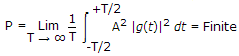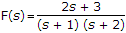# Electronics and Communication Engineering - Signals and Systems

Exercise : Signals and Systems - Section 1
41.
A signal g(t) = A then g(t) is a
energy signal
power signal
neither energy nor power signal
insufficient data
Explanation:.

42.
The Fourier series of an odd periodic function contains
odd harmonics only
even harmonics only
cosine harmonics only
sine harmonics only
Explanation:

For an odd function f(- x) = - f(x) Hence, only sine terms.

43.
If, the terms in f(t) will have
e-t and e-2t
et and e2t
te-t and te-2t
none of the above
Explanation:

(s + 1) term will give e-t and (s + 2) term will give e-2t .

44.
An impulse function consist of
pure dc
pure a.c
entire frequency range with constant phase
infinite bandwidth with linear phase vaariations
Explanation:

Because F.T. of δ(t) = 1, 1 is a Constant function ranging from -∞ to +∞.

45.
As per time displacement theorem in Laplace transformation, displacement in the time domain by T becomes
division by s in the s domain
division by e-sT in the s domain
multiplication by s in the s domain
multiplication by e-sT in the s domain